##### The Japanese Bonsai specialist
Direct order Contact Help / Services Newsletter# Carving tool for jin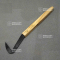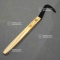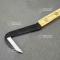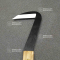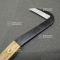ref. : 11883

19,00

Available quantity : 8Order

###### Description

This tool is for carving back wood bark on branches and trunks and creating deadwood: jin and shari. The blade is for a right-handed person. Japanese forged manufacture. Length: 200 mm. The blade’s special shape makes for precise, accurate work.

#carving 3.7 #shari 3.5 #tool 3.4 #tools 2.5 #blade 2.5 #manufacture 2.1 #japanese 1.8 #accurate 1.8 #creating 1.8 #deadwood 1.8

Formule
(( ROUND((CHAR_LENGTH(b.article_nom)-CHAR_LENGTH(REPLACE(b.article_nom, 'carving', '')))/LENGTH('carving')) + ROUND((CHAR_LENGTH(b.article_description)-CHAR_LENGTH(REPLACE(b.article_description, 'carving', '')))/LENGTH('carving')) ) * 3.7) + (( ROUND((CHAR_LENGTH(b.article_nom)-CHAR_LENGTH(REPLACE(b.article_nom, 'tool', '')))/LENGTH('tool')) + ROUND((CHAR_LENGTH(b.article_description)-CHAR_LENGTH(REPLACE(b.article_description, 'tool', '')))/LENGTH('tool')) ) * 3.4) + (( ROUND((CHAR_LENGTH(b.article_nom)-CHAR_LENGTH(REPLACE(b.article_nom, 'blade', '')))/LENGTH('blade')) + ROUND((CHAR_LENGTH(b.article_description)-CHAR_LENGTH(REPLACE(b.article_description, 'blade', '')))/LENGTH('blade')) ) * 2.5) + (( ROUND((CHAR_LENGTH(b.article_nom)-CHAR_LENGTH(REPLACE(b.article_nom, 'manufacture', '')))/LENGTH('manufacture')) + ROUND((CHAR_LENGTH(b.article_description)-CHAR_LENGTH(REPLACE(b.article_description, 'manufacture', '')))/LENGTH('manufacture')) ) * 2.1) + (( ROUND((CHAR_LENGTH(b.article_nom)-CHAR_LENGTH(REPLACE(b.article_nom, 'accurate', '')))/LENGTH('accurate')) + ROUND((CHAR_LENGTH(b.article_description)-CHAR_LENGTH(REPLACE(b.article_description, 'accurate', '')))/LENGTH('accurate')) ) * 1.8) + (( ROUND((CHAR_LENGTH(b.article_nom)-CHAR_LENGTH(REPLACE(b.article_nom, 'japanese', '')))/LENGTH('japanese')) + ROUND((CHAR_LENGTH(b.article_description)-CHAR_LENGTH(REPLACE(b.article_description, 'japanese', '')))/LENGTH('japanese')) ) * 1.8) + (( ROUND((CHAR_LENGTH(b.article_nom)-CHAR_LENGTH(REPLACE(b.article_nom, 'branches', '')))/LENGTH('branches')) + ROUND((CHAR_LENGTH(b.article_description)-CHAR_LENGTH(REPLACE(b.article_description, 'branches', '')))/LENGTH('branches')) ) * 1.8) + (( ROUND((CHAR_LENGTH(b.article_nom)-CHAR_LENGTH(REPLACE(b.article_nom, 'creating', '')))/LENGTH('creating')) + ROUND((CHAR_LENGTH(b.article_description)-CHAR_LENGTH(REPLACE(b.article_description, 'creating', '')))/LENGTH('creating')) ) * 1.8) + (( ROUND((CHAR_LENGTH(b.article_nom)-CHAR_LENGTH(REPLACE(b.article_nom, 'deadwood', '')))/LENGTH('deadwood')) + ROUND((CHAR_LENGTH(b.article_description)-CHAR_LENGTH(REPLACE(b.article_description, 'deadwood', '')))/LENGTH('deadwood')) ) * 1.8) + (( ROUND((CHAR_LENGTH(b.article_nom)-CHAR_LENGTH(REPLACE(b.article_nom, 'precise', '')))/LENGTH('precise')) + ROUND((CHAR_LENGTH(b.article_description)-CHAR_LENGTH(REPLACE(b.article_description, 'precise', '')))/LENGTH('precise')) ) * 1.7)

## Secure payment## Delivery

Our logistic partners :04 74 55 23 48
Pépinière MAILLOT-BONSAÏ
Le Bois Frazy
01990 RELEVANT - FRANCE
on appointment